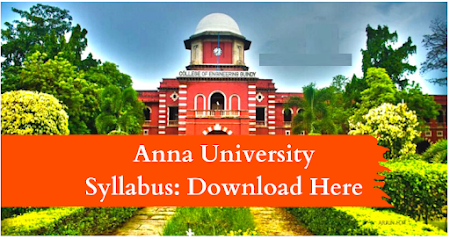### EC8451    ELECTROMAGNETIC FIELDS   L T P C 4 0 0 4

OBJECTIVES:  To gain conceptual and basic mathematical understanding of electric and magnetic fields in free space and in materials  To understand the coupling between electric and magnetic fields through Faraday's law, displacement current and Maxwell's equations  To understand wave propagation in lossless and in lossy media  To be able to solve problems based on the above concepts

UNIT I      INTRODUCTION                                                                                                        12 Electromagnetic model, Units and constants, Review of vector algebra, Rectangular, cylindrical and spherical coordinate systems, Line, surface and volume integrals, Gradient of a scalar field, Divergence of a vector field, Divergence theorem, Curl of a vector field, Stoke's theorem, Null identities, Helmholtz's theorem

UNIT II    ELECTROSTATICS                                                                                                     12 Electric field, Coulomb's law, Gauss's law and applications, Electric potential, Conductors in static electric field, Dielectrics in static electric field, Electric flux density and dielectric constant, Boundary conditions, Capacitance, Parallel, cylindrical and spherical capacitors, Electrostatic energy, Poisson's and Laplace's equations, Uniqueness of electrostatic solutions, Current density and Ohm's law, Electromotive force and Kirchhoff's voltage law, Equation of continuity and Kirchhoff's current law

UNIT III   MAGNETOSTATICS                                                                                                   12 Lorentz force equation, Law of no magnetic monopoles, Ampere's law, Vector magnetic potential, Biot-Savart law and applications, Magnetic field intensity and idea of relative permeability, Magnetic circuits, Behaviour of magnetic materials, Boundary conditions, Inductance and inductors, Magnetic energy, Magnetic forces and torques

UNIT IV   TIME-VARYING FIELDS AND MAXWELL's EQUATIONS                                12 Faraday's law, Displacement current and Maxwell-Ampere law, Maxwell's equations, Potential functions, Electromagnetic boundary conditions, Wave equations and solutions, Time-harmonic fields

UNIT V   PLANE ELECTROMAGNETIC WAVES                                                                    12 Plane waves in lossless media, Plane waves in lossy media (low-loss dielectrics and good conductors), Group velocity, Electromagnetic power flow and Poynting vector, Normal incidence at a plane conducting boundary, Normal incidence at a plane dielectric boundary

TOTAL:60 PERIODS
OUTCOMES: By the end of this course, the student should be able to:  Display an understanding of fundamental electromagnetic laws and concepts  Write Maxwell's equations in integral, differential and phasor forms and explain their physical meaning  Explain electromagnetic wave propagation in lossy and in lossless media  Solve simple problems requiring estimation of electric and magnetic field quantities based on these concepts and laws

TEXT BOOKS:
1. D.K. Cheng, Field and wave electromagnetics, 2nd ed., Pearson (India), 1989 (UNIT I, II,III IV,V) 2. W.H. Hayt and J.A. Buck, Engineering electrmagnetics, 7th ed., McGraw-Hill (India), 2006 (UNIT I-V)

REFERENCES
1. D.J. Griffiths, Introduction to electrodynamics, 4th ed., Pearson (India), 2013
2. B.M. Notaros, Electromagnetics, Pearson: New Jersey, 2011
3. M.N.O. Sadiku and S.V. Kulkarni, Principles of electromagnetics, 6th ed., Oxford (Asian Edition), 2015